One of the things we learn in mathematics is the Pythagorean theorem. Even if there is a side with an unknown length, you can use the Pythagorean theorem to calculate its length.

In order to understand the Pythagorean theorem, you need to have already learned about square roots. As long as you understand square roots, you can use the Pythagorean theorem.

As for the Pythagorean theorem, you should memorize the formula. The Pythagorean theorem is a formula that is frequently used in mathematics, and if you do not memorize the formula, you will not be able to solve calculation problems. Also, in special triangles, it is easier to find the lengths of the sides.

So we will explain how to use the Pythagorean theorem to solve math calculation problems.

## What Is the Pythagorean Theorem: Right Triangles and Side Lengths

First of all, what is the Pythagorean theorem? The Pythagorean theorem is a formula proved by Pythagoras, an ancient Greek mathematician.

In the Pythagorean theorem, we must always use a right triangle. In the case of a right triangle, the relationship between the hypotenuse and other sides is as follows.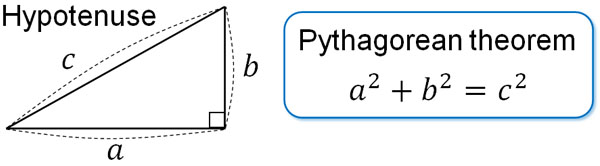In the case of right triangles, the Pythagorean theorem holds for all shapes. Since it is a simple formula, the Pythagorean theorem is used in many calculations.

### Pythagorean Theorem for Calculating Unknown Side Lengths

Why is the Pythagorean theorem used so often? It is because we can calculate the length of unknown sides.

For example, what is the length of the following side $a$?Using the Pythagorean theorem, we can create the following equation.

• $8^2=a^2+4^2$

Solving this expression, we get the following.

$8^2=a^2+4^2$

$64=a^2+16$

$a^2=48$

$a=4\sqrt{3}$

There are two answers for $a^2=48$: $±4\sqrt{3}$. However, in the case of shapes, the answer can never be negative. Therefore, there is only one answer, $a=4\sqrt{3}$ cm.

-Calculating the Lengths of Hypotenuse and Other Sides

If you know the two side lengths of a right triangle, you can use the Pythagorean theorem to calculate the side lengths. By using the hypotenuse and other sides, we can get the side lengths.

Be careful not to mistake the position of the hypotenuse. The following is a hypotenuse.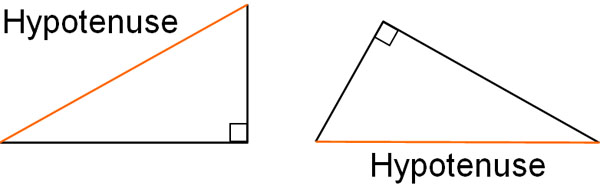When using the Pythagorean theorem, it must be a right triangle. In this case, the side opposite the right angle is the hypotenuse.

### Proof of the Pythagorean Theorem

Why does the Pythagorean theorem work? When solving math problems, we do not have to prove the Pythagorean theorem. However, it is important as knowledge to understand why the formula is valid. So, we will prove that the Pythagorean theorem is satisfied.

First, suppose we have the following triangle.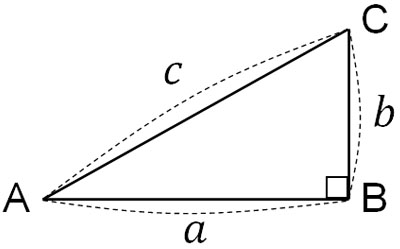For this triangle, make a regular quadrilateral with AC as a side. The length of the side is $c$ and is as follows.Next, three triangles congruent to △ABC are placed around the regular quadrilateral ACDE as follows.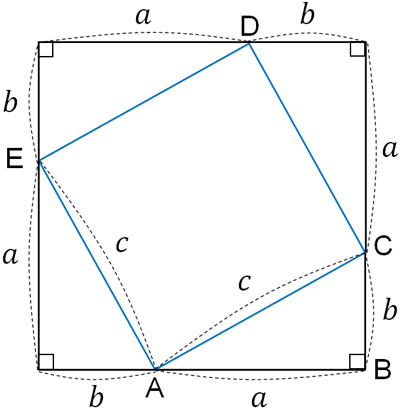What would be the equation to get the area of the square ACDE? Since the length of the side is $c$, the area of the square ACDE is $c^2$.

The area of the square ACDE can also be calculated by another method. By placing △ABC around the square ACDE, we have created a large square. Subtracting the four △ABCs from the area of the large square, we can get the area of the square ACDE.

The area of the large square is $(a+b)^2$. Also, the area of △ABC is $\displaystyle\frac{1}{2}ab$. Therefore, the area of the square ACDE can be given by the following equation.

• $(a+b)^2-\displaystyle\frac{1}{2}ab×4$

So by using the area $c^2$ of the square ACDE, we can create the following expression.

• $c^2=(a+b)^2-\displaystyle\frac{1}{2}ab×4$

Solving this equation, we get $c^2=a^2+b^2$. Thus, we are able to prove the Pythagorean theorem.

## Pythagorean Theorem Used for Specially Shaped Triangles

The Pythagorean theorem can be used in all right triangles. Some triangles have a special shape.

Even though they are special triangles, there are many math problems that need to be solved using special triangles and the Pythagorean theorem. So let’s understand not only the basics of the Pythagorean theorem but also the Pythagorean theorem using specially shaped triangles.

Specifically, the following right-angled triangles fall under the category of special shapes.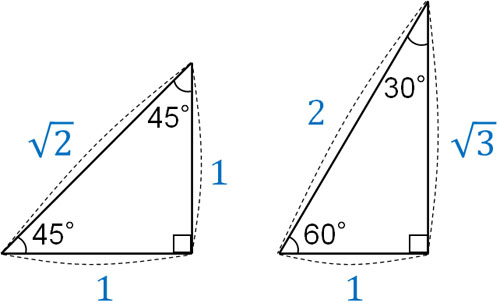It is important to note that the ratio of the side lengths is fixed in these right triangles.

For a right triangle with two angles of 45°, the ratio of the sides is $1:1:\sqrt{2}$. On the other hand, for a right triangle with angles of 30° and 60°, the ratio of the sides is $2:1:\sqrt{3}$.

### Isosceles Right Triangle: A Right Triangle with Two Angles of 45°

This side ratio is frequently used in mathematical calculations. If you don’t remember the ratios of the sides, you won’t be able to solve mathematical problems. Therefore, you must memorize the ratios of the sides. For example, what is the length of the following side $a$?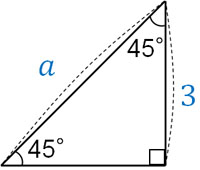For a right triangle with two angles of 45°, the ratio of the corresponding sides in the above figure is $1:\sqrt{2}$. Therefore, we can create the following proportional relationship.

• $1:\sqrt{2}=3:a$

Solving this equation, we get $a=3\sqrt{2}$. By using the ratio of the sides, we can find the side lengths. As mentioned above, you need to remember the side ratios in order to solve the problem.

### Right Triangles with 30° and 60° Angles

On the other hand, how do we think about right triangles with angles of 30° and 60°? The idea is the same as before. By using the ratio of the sides, we can calculate the side lengths.

For example, what is the length of $a$ in the following right triangle?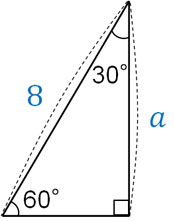The side ratio is $2:1:\sqrt{3}$. Of these, the corresponding side ratio to the above figure is $2:\sqrt{3}$. Therefore, we can create the following proportional relationship.

• $2:\sqrt{3}=8:a$

Solving this equation, we get $a=4\sqrt{3}$. Check which side is the corresponding side of $2:\sqrt{3}$ before making the proportional relationship.

## Calculating the Diagonal Length of a Cube: Calculating Space Figures

Pythagorean theorem is not only used for plane figures but also for space figures. The reason why the Pythagorean theorem is often used in architecture and structural mechanics is that it allows us to calculate the length of unknown sides in space figures.

For example, what is the diagonal length of the following cuboid?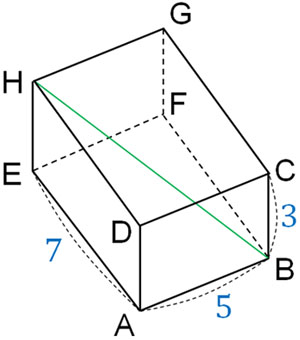To do this calculation, draw a line as shown below.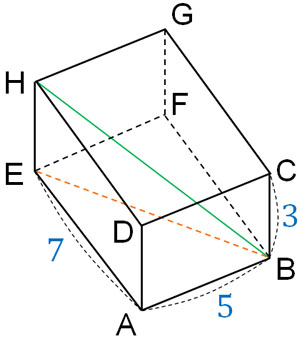By using the Pythagorean theorem, we can calculate the number EB2. It is as follows.

• $EB^2=7^2+5^2=74$

Then, what is the length of side BH? Since △BEH is a right triangle, we can use the Pythagorean theorem. Also, since we know the number EB2, we can calculate the length of side BH as follows.

• $BH^2=74+3^2=83$
• $BH=\sqrt{83}$

Thus, we can calculate the length of the side BH as $\sqrt{83}$.

## Exercise: Calculations Using the Pythagorean Theorem

Q1: For the following triangle, calculate the volume of the three-dimensional object formed when it is rotated by one rotation around the axis of side AB.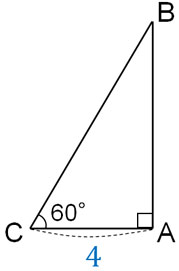First, let’s calculate the length of AB because AB is the height of the 3D figure. By using the side ratio of the special triangle, we can create the following proportional relationship.

• $1:\sqrt{3}=4:AB$

Solving for this gives us $AB=4\sqrt{3}$. If you can calculate AB, you can solve this problem in the same way as the problem of the solid of revolution. If we make one rotation around AB, we can create the following three-dimensional figure.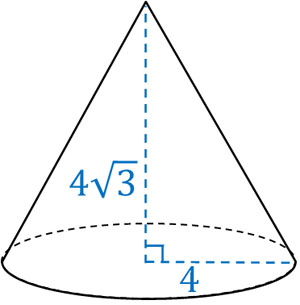Such a cone is created, and since we know the radius and height, we can calculate the volume.

• $4×4×π×4\sqrt{3}×\displaystyle\frac{1}{3}=\displaystyle\frac{64\sqrt{3}}{3}$

Thus, we are able to calculate the volume of the cone as $\displaystyle\frac{64\sqrt{3}}{3}$.

## Calculating the Length of an Unknown Side

In figures, even if there is an unknown side length, you can find out the length by calculating it. This can be done without directly measuring the length. The Pythagorean theorem is often used in this case.

For right triangles, the Pythagorean theorem can always be used. If you know the lengths of two sides, you can calculate the lengths of the other side. In the case of a right triangle with a special shape, if you know the length of one side, you can calculate the lengths of all sides.

When using the Pythagorean theorem, try to find a right triangle. Then check which one is the hypotenuse, and you can solve the problem.

In this section, we proved why the Pythagorean theorem is true. However, what is more important is to be able to use the Pythagorean theorem to solve problems. So try to use the Pythagorean theorem to calculate the side lengths of plane and space figures.